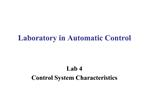DownloadDownload PresentationLaboratory in Automatic Control

# Laboratory in Automatic Control

Download Presentation## Laboratory in Automatic Control

- - - - - - - - - - - - - - - - - - - - - - - - - - - E N D - - - - - - - - - - - - - - - - - - - - - - - - - - -
##### Presentation Transcript

1. Laboratory in Automatic Control Lab 4 Control System Characteristics

2. Max and Min Function Syntax C=max(A) D=min(A) MATLAB code

3. Residue Function Syntax [r,p,k]=residue(num,den) MATLAB code

4. Flow Control Syntax for variable=expression statements end MATLAB code

5. Flow Control MATLAB code

6. Flow Control Syntax if expression statements end MATLAB code

7. Flow Control Syntax if expression 1 statements 1 elseif expression 2 statements 2 elseif expression N-1 statements N-1 else statements N end

8. Flow Control MATLAB code

9. Flow Control Syntax switch switch_expr case case_expr statement, ..., statement case {case_expr1, case_expr2, case_expr3, ...} statement, ..., statement otherwise statement, ..., statement end

10. Flow Control MATLAB code

11. Flow Control Example MATLAB code

12. Flow Control

13. Flow Control MATLAB code

14. Flow Control

15. Lab 4 Homework P1. Consider the transfer function (without feedback) when the input is a unit step, the desired steady-state value of the output is one. Using the MATLAB step function, show that the steady-state error to a unit step input is 0.8. ( )

16. Lab 4 Homework P2. Consider the closed-loop control system shown in Figure 1. Develop a MATLAB script to assist in the search for a value of k so that the percent over-shoot to a unit step input is greater than 1 %, but less than 10%. The script should compute the closed-loop transfer function, , and generate the step response. Verify graphically that the steady-state error to a unit step input is zero. (Hint: search k within [1, 5], )